# Sample Data: Ozarks Karst

Locations of springs and sinkholes in the Ozark Plateaus Physiographic Province (Ozarks) in Arkansas

## Details

Locations of springs and sinkholes in the Ozark Plateaus Physiographic Province (Ozarks) in Arkansas.

## Examples

### Basic Examples (2)

Retrieve the data:

 In:=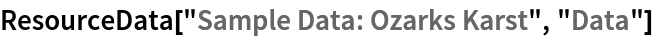Out=Summary of the spatial point data:

 In:=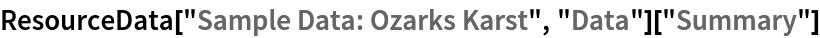Out=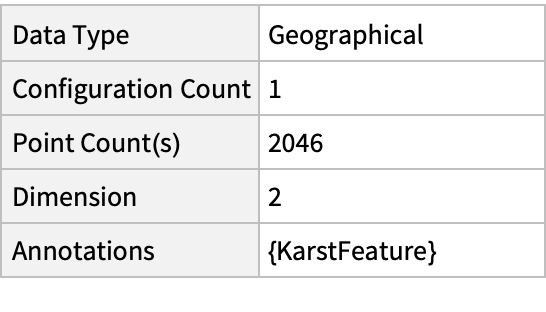### Visualizations (2)

Plot the spatial point data:

 In:=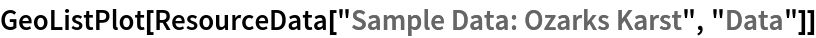Out=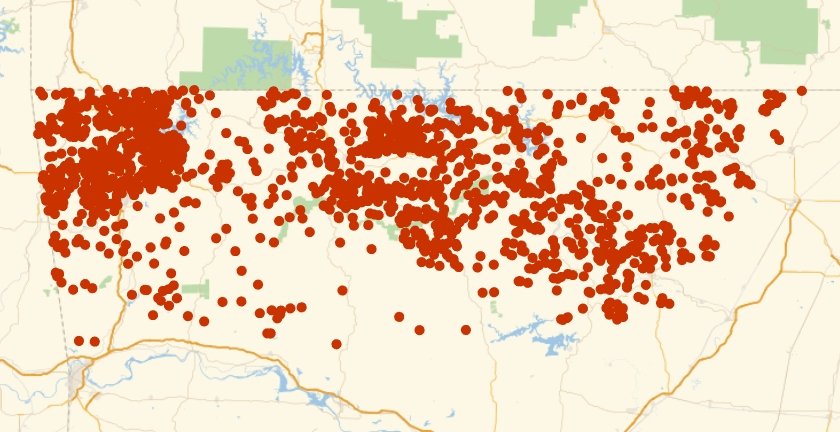Visualize the data with annotations:

 In:=Out=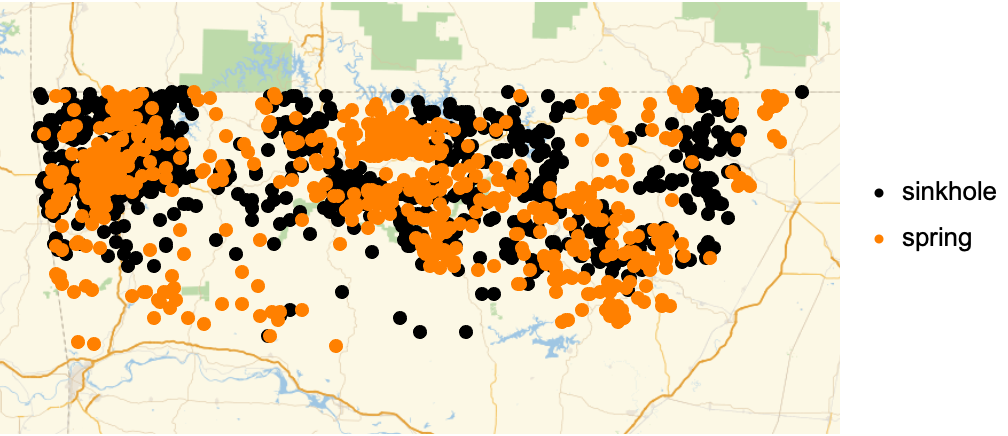### Analysis (3)

Compute probability of finding a point within given radius of an existing point - NearestNeighborG is the CDF of the nearest neighbor distribution:

 In:=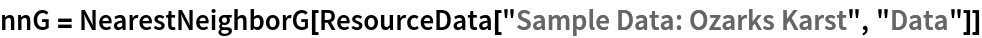Out=In:=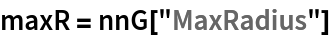Out=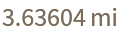In:=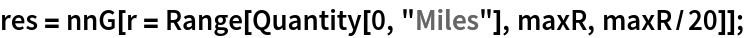In:=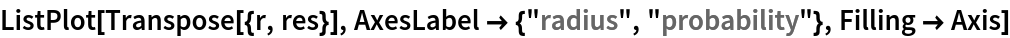Out=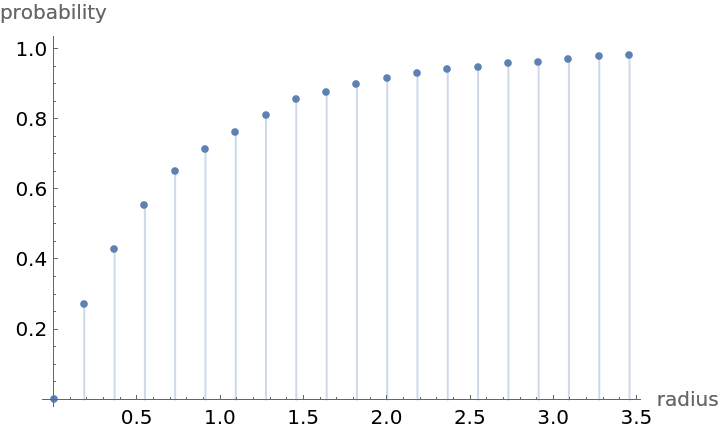Mean distance between a typical point and its nearest neighbor (for positive support distribution can be approximated via a Riemann sum of 1-CDF):

 In:=In:=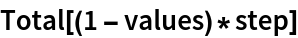Out=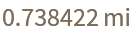Test for complete spatial randomness:

 In:=Out=Gosia Konwerska, "Sample Data: Ozarks Karst" from the Wolfram Data Repository (2021)